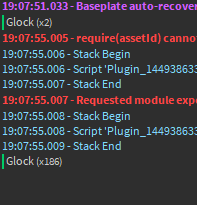# How to randomize according to number? (chances)

Hello! so, I was wondering sorry about the short topic, but how to make for example

``````local Items = {
{Name="Fal",RandomNumber=0.1} -- Complicated for it to shows
{Name="Glock",RandomNumber=10} -- Easy for it to shows
}
``````

I guess you know what I mean right? That it goes according to a random number, like apocalypse rising, most of times you found bad guns, but sometimes you found like, op guns, I’m not asking for code, just asking for an example of how can I make it?

3 Likes

There is a function called math.random(m, n) which generates a pseudo-random number. You can specify the range of the function, from a minimum of `m` to a maximum of `n`. You can use this to create randomness.

Hey, so I know there’s a function,
like

``````print(math.random(1,2)) -- example: 1
``````

but this is not what i’m trying to make, it’s according to anumber, how can I make it?

1 Like

You can make a dictionary with different values inside of them, sort of like making simulator eggs and hatching a variety of pets:

``````local Items = {
BadGun = {Name = "BadGun", Rarity = 100}, -- The higher the rarity, the more it spawns
GoodGun = {Name = "BadGun", Rarity = 10},
BestGun = {Name = "BadGun", Rarity = 1}
}
``````

You would then loop through and add up all the rarity values

``````local UniversalRarity = 0

for i, v in pairs(Items) do
UniversalRarity = UniversalRarity + v.Rarity
end
``````

Then you would assign locations on where the guns would spawn and loop through all the locations:

``````local Locations = -- Table of all the locations where the guns will spawn, or random locations

local UniversalRaritySubtract = UniversalRarity -- Were cloning the value, because this one will be changed
local Index = 1
for i, Location in pairs(Locations) do
local Chance = math.random(1, UniversalRarity)
UniversalRaritySubtract =  UniversalRaritySubtract - Chance
Index = Index + 1
if  UniversalRaritySubtract <= Items[Index].Rarity then
print(Items[Index].Name) -- prints out the chosen gun
end
end
``````

The final code would look something like this:

``````local Items = {
GoodGun = {Name = "BadGun", Rarity = 10},
BestGun = {Name = "BadGun", Rarity = 1}
}

local UniversalRarity = 0

for i, v in pairs(Items) do
UniversalRarity = UniversalRarity + v.Rarity
end

local Locations = -- Table of all the locations where the guns will spawn, or random locations

local UniversalRaritySubtract = UniversalRaritychanged
local Index = 1
for i, Location in pairs(Locations) do
local Chance = math.random(1, UniversalRarity)
UniversalRaritySubtract =  UniversalRaritySubtract - Chance
Index = Index + 1
if  UniversalRaritySubtract <= Items[Index].Rarity then
print(Items[Index].Name)
end
end
``````
3 Likes

Hey there! A way to do what you’re asking—loot tables—can be similarly achieved like so:

``````-- We declare the loot "table" array.
local items = {
-- Every element in the array is an object with a "weight" key indicating its chance. The value of weight is relative.
{
name = "Fal",
weight = 10,
},
{
name = "Glock",
weight = 90,
},
};

-- A function that, when given a loot table, returns the sum of its weights.
local function getSumOfWeightsInLootTable(lootTable)
local sum = 0;
for _, item in ipairs(lootTable) do
sum = sum + item.weight;
end
return sum;
end

-- A function that, when given a loot table, returns a random item.
local function getRandomItemFromLootTable(lootTable)
local randomValue = math.random(0, getSumOfWeightsInLootTable(lootTable));
for _, item in ipairs(lootTable) do
if randomValue <= item.weight then
return item.name;
else
randomValue = randomValue - item.weight;
end
end
end
``````

Realistically, in a larger project or a project that should be built for maintainability (every and all projects that aren’t a 5-minute prototype), this would be abstracted to being a user-implemented service in some module. Also, this method should be tweaked to your needs. Instead of returning the name in your quick example, it would return the gun model or something similar for more concise and practical use.

3 Likes

So how does it works?
So you have the sum

(example)
10 in this case, because fal and glock returns 10
so

You’re doing math.random(getSumOfWeightInLootTable(lootTable))
But, math.random required 2 parametters right?

EDIT:Is always returing Glock?

Good question! Not quite, as both parameters are optional! This means that when supplied with no arguments, `math.random()` returns a number in the range “[0, 1)” (that is to mean any number above one and up to one, inclusively). If given one parameter, `math.random(m)` it returns a number in the range “[1, m]” (one inclusively to m inclusively). Finally, when given two parameters, `math.random(m, n)` returns a value in the range “[m, n]”.

This does mean, actually, that my decimals here won’t do much good. I’ve updated the data in the loot table to fix this.

1 Like

Also, Fast fast fast question, what’s difference between LOCAL FUNCTION and FUNCTION?I’d be glad to help; never be afraid to ask! It’s a matter of a variable’s scope. Let me explain:

Consider the following:

``````
local function greet()
local function getGreeting()
return "Hello";
end

function getNoun()
return "World";
end

print(("%s, %s!"):format(getGreeting(), getNoun()));
end

print(getGreeting, ", ", getNoun);
-- Prints "nil, nil"

greet();
-- Prints "Hello, World!"

print(getGreeting, ", ", getNoun);
-- Prints "nil, function 0x...." (it is still accessible!)
``````

This illustrates that global functions, once declared, are accessible from anywhere in the script whereas local functions are only accessible in the scope they’re declared. This is the same for variables. It is highly recommended you use the local scope within conceivably every scenario, especially in production code.